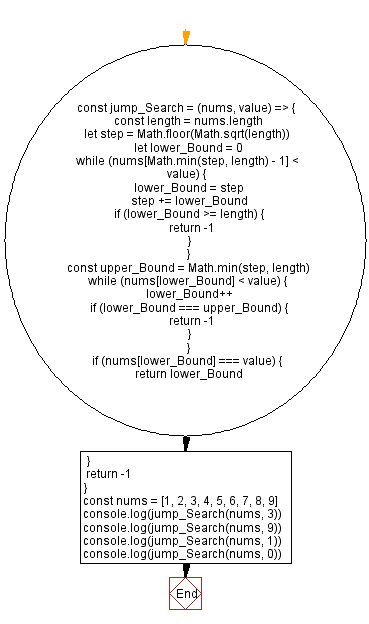# JavaScript - Jump search algorithm

## JavaScript Searching Algorithm: Exercise-4 with Solution

Write a JavaScript program to find an element in a given sorted array of elements using Jump Search.

From Wikipedia, in computer science, a jump search or block search refers to a search algorithm for ordered lists. It works by first checking all items Lkm, where κ ∈ Ν and m is the block size, until an item is found that is larger than the search key. To find the exact position of the search key in the list a linear search is performed on the sublist L[(k-1)m, km].

Test Data:
([1, 2, 3, 4, 5, 6, 7, 8, 9], 3) -> 2
([1, 2, 3, 4, 5, 6, 7, 8, 9], 9) -> 8
([1, 2, 3, 4, 5, 6, 7, 8, 9], 1) -> 0
([1, 2, 3, 4, 5, 6, 7, 8, 9], 0) -> -1

Sample Solution:

JavaScript Code:

``````const jump_Search = (nums, value) => {
const length = nums.length
let step = Math.floor(Math.sqrt(length))
let lower_Bound = 0
while (nums[Math.min(step, length) - 1] < value) {
lower_Bound = step
step += lower_Bound
if (lower_Bound >= length) {
return -1
}
}
const upper_Bound = Math.min(step, length)
while (nums[lower_Bound] < value) {
lower_Bound++
if (lower_Bound === upper_Bound) {
return -1
}
}
if (nums[lower_Bound] === value) {
return lower_Bound
}
return -1
}
const nums = [1, 2, 3, 4, 5, 6, 7, 8, 9]
console.log(jump_Search(nums, 3))
console.log(jump_Search(nums, 9))
console.log(jump_Search(nums, 1))
console.log(jump_Search(nums, 0))
```
```

Sample Output:

```2
8
0
-1
```

Flowchart:Live Demo:

See the Pen javascript-searching-algorithm-exercise-4 by w3resource (@w3resource) on CodePen.

* To run the code mouse over on Result panel and click on 'RERUN' button.*

Improve this sample solution and post your code through Disqus

Previous: Ternary search algorithm.
Next: Interpolation algorithm.

What is the difficulty level of this exercise?

Test your Programming skills with w3resource's quiz.

﻿